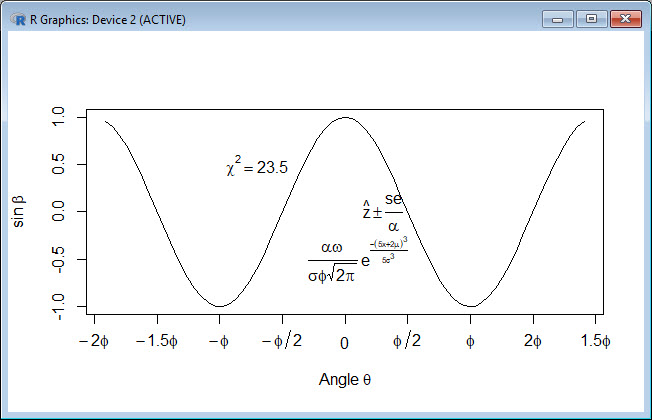In Blog 22, let’s see how to create mathematical expressions for your graph.
Mathematical expressions on graphs are made possible through `expression(paste())` and `substitute()`. If you need mathematical expressions as axis labels, switch off the default axes and include Greek symbols by writing them out in English. You can create fractions through the `frac()` command. Note how we obtain the plus or minus sign through the syntax: `%+-%`
Here is a nice example. Let’s create a set of 71 values from – 6 to + 6. These values are the horizontal axis values.
`x <- seq(-6, 6, len = 71)`
Now we plot a cosine function using a continuous curve (using `type="l"`) while suppressing the x axis using the syntax: `xaxt="n"`
`plot(x, cos(x),type="l",xaxt="n", xlab=expression(paste("Angle ",theta)), ylab=expression("sin "*beta))`
where we have inserted relevant mathematical text for the axis labels using `expression(paste())`. Here is the graph so far:Now we create a horizontal axis to our own specifications, including relevant labels:
`axis(1, at = c(-2*pi, -1.5*pi, -pi, -pi/2, 0, pi/2, pi, 1.5*pi, 2*pi), lab = expression(-2*phi, -1.5*phi, -phi, -phi/2, 0, phi/2, phi, 2*phi, 1.5*phi))`Let’s put in some mathematical expressions, centred appropriately. The first argument within each `text()` function gives the value along the horizontal axis about which the text will be centred.
```text(-0.7*pi,0.5,substitute(chi^2=="23.5")) text(0.1*pi, -0.5, expression(paste(frac(alpha*omega, sigma*phi*sqrt(2*pi)), " ", e^{frac(-(5*x+2*mu)^3, 5*sigma^3)}))) text(0.3*pi,0,expression(hat(z) %+-% frac(se, alpha)))```
Here is our graph, complete with mathematical expressions:That wasn’t so hard! In Blog 23 we will look at further plotting techniques in R.
See you later!
David

#### Annex: R codes used

```# Create a set of 71 horizontal axis values.
x <- seq(-6, 6, len = 71)

# Plot a cosine function.
plot(x, cos(x),type="l",xaxt="n", xlab=expression(paste("Angle ",theta)), ylab=expression("sin "*beta))

# Custimise a horizontal axis.
axis(1, at = c(-2*pi, -1.5*pi, -pi, -pi/2, 0, pi/2, pi, 1.5*pi, 2*pi), lab = expression(-2*phi, -1.5*phi, -phi, -phi/2, 0, phi/2, phi, 2*phi, 1.5*phi))

# Add a few mathematical expressions.
text(-0.7*pi,0.5,substitute(chi^2=="23.5"))
text(0.1*pi, -0.5, expression(paste(frac(alpha*omega, sigma*phi*sqrt(2*pi)), " ", e^{frac(-(5*x+2*mu)^3, 5*sigma^3)})))
text(0.3*pi,0,expression(hat(z) %+-% frac(se, alpha)))
```